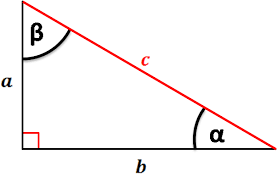# Java Program to Calculate Hypotenuse Of Triangle | Programs

Java program to calculate hypotenuse of a triangle – In this specific article, we will learn the multiple ways to calculate the hypotenuse of a triangle in Java programming – also check area of triangle java code.

Suitable examples and sample programs have been included in order to make you understand simply.

• Using Standard Method
• Using Command-Line Arguments
• Using Scanner Class
• Using Static Method
• Using Separate Class

A right triangle is a triangle where one of the angles is a right angle or is equal to 90 degrees. Hence, it is obvious that the sum of the other two angles is also equal to 90 degrees.

The side which is opposite to that of the right angle is known as the hypotenuse.As you can see, the side c is opposite to the right angle. Hence, c is the hypotenuse of this triangle. According to the Pythagoras theorem,

a^2 + b^2 = c^2.

In words, the square of the hypotenuse is equal to the sum of the squares of both the sides. This is how you generally calculate the hypotenuse of a right triangle.

Thus, the multiple ways to calculate the hypotenuse of a right triangle in Java programming are as follows:

Using Standard Method

Calculating the hypotenuse of a triangle is very simple. To do this, we’ll need the length of two side (adjacent and opposite). This value can be assigned to the variable in the code itself. After getting the inputs, hypotenuse can be calculated as square-root of sum of square both the sides.

To find the square-root we can make use of the predefined method sqrt() from the Math package as follows:

This resultant hypotenuse can then be displayed on our output console screen.

Output:

## Using Command Line Arguments

In the previous method, the inputs were given in the code itself. So, for a different set of inputs, the change must be made in the code which is not very convenient.

Instead of that, we can give inputs as command line arguments for the code.

Here, while giving the run command, after the name of code to be run, the same number of arguments can be passed as required in the input with space between each of them.

These inputs are of string type and need to be converted into double type by parsing them as follows:

After gathering inputs, the same calculation as in the previous method is to be made to get out resultant hypotenuse.

Output1:

Using Scanner Class

Another way of taking inputs at runtime is by making use of Scanner class in Java. With the help of Scanner class, any primitive datatype inputs can be read at runtime.

So, we read the lengths of two sides of the triangle with Scanner class as follows:

Hypotenuse is nothing but the square-root of sum of square of the two sides by Pythagoras theorem. It can be calculated by making use of sqrt() method of the Math package as follows:

hypotenuse = Math.sqrt((side1*side1)+(side2*side2));

Using Static Method

Static method can be made use of in this problem so that, the main logic of the code for calculating the length of hypotenuse can be made reusable.

By doing so, in future if somewhere else in the code, we would like to make use of the same logic we can just do by calling this method instead of rewriting the statements.

Here, main method reads the length of two sides as input with the help of Scanner class. Then, another static method (hypoCal) is called and the inputs gathered is passed as parameters.

This static method (hypoCal), calculates the length of hypotenuse by making use of both sqrt() and pow() method from the Math package as follows:

Math.sqrt(Math.pow(s1, 2) + Math.pow(s2, 2));

This value is returned by the static method(hypoCal) which is our resultant output.

Output1:

x

## Java Program to Add Two Matrices – 4 Ways | Programs

Java program to add two matrices – The following Java Code will let you know ...